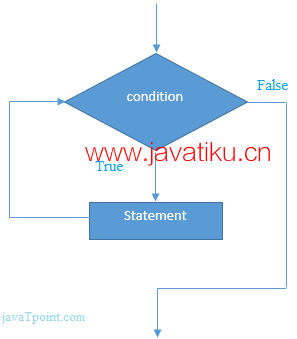# C++教程-C++while循环## C++ while循环

C++的while循环用于在程序中重复执行一部分代码。如果迭代次数不固定，建议使用while循环而不是for循环。

``````while (condition) {
// 需要执行的代码
}``````### C++ while循环示例：

``````#include <iostream>
using namespace std;

int main() {
int i = 1;
while (i <= 10) {
cout << i << "\n";
i++;
}
return 0;
}``````

``````1
2
3
4
5
6
7
8
9
10``````

### C++嵌套while循环示例：

``````#include <iostream>
using namespace std;

int main() {
int i = 1;
while (i <= 3) {
int j = 1;
while (j <= 3) {
cout << i << " " << j << "\n";
j++;
}
i++;
}
return 0;
}``````

``````1 1
1 2
1 3
2 1
2 2
2 3
3 1
3 2
3 3``````

### C++无限while循环示例：

``````#include <iostream>
using namespace std;

int main() {
while (true) {
cout << "无限while循环";
}
return 0;
}``````

``````无限while循环